{{announcement.body}}
{{announcement.title}}

# Big-O Ambiguity

DZone 's Guide to

# Big-O Ambiguity

### Here's a brief crash course on Big-O notation in relation to Big-Ω and Big-Θ notation, as well as a refresher on its usefulness.

· Performance Zone ·
Free Resource

Comment (0)

Save
{{ articles.views | formatCount}} Views

## Back to University

Most people talk about Big-O notation when it comes to runtime and space complexity. But when you recall your first year at university, most probably you had an Algorithm and Data Structure course when you have learned not only Big-O notation, but also Big-Ω (Omega) and Big-Θ (Theta). Here is a very brief definition:

• if f(n) is O(g(n)), it means that f(n) grows asymptotically no faster than g(n)
• if f(n) is Ω(g(n)), it means that f(n) grows asymptotically no slower than g(n)
• if f(n) is Θ(g(n)), it means that f(n) grows asymptotically at the same rate as g(n)

In other words, O(g(n)) is an upper bound which means that from certain point (n0 on the graph below), c*g(n) is never below the function f(n) which we are analyzing (don’t worry about c — it is just a constant).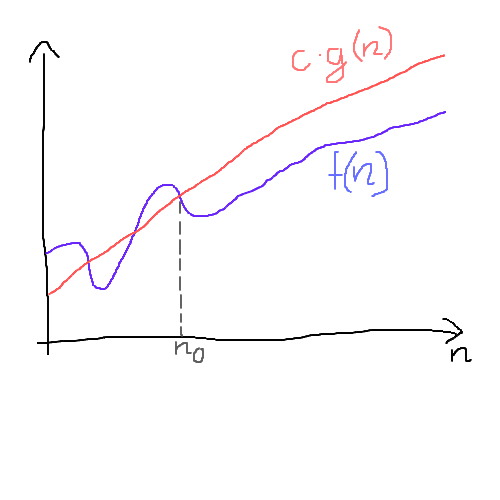Ω(g(n)) is a lower bound which means that from the certain point (n0), c*g(n) is never above our function f(n).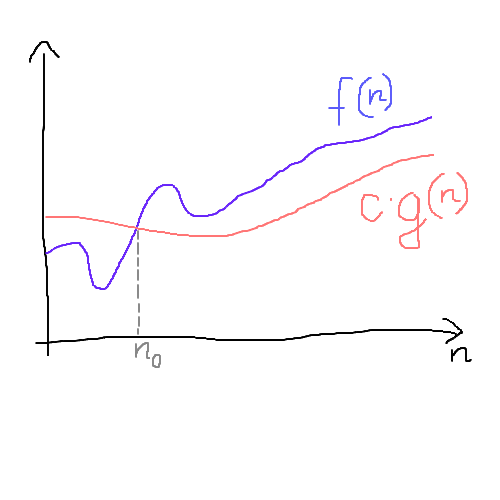Θ(g(n)) means that function g(n) grows exactly in the same asymptotic way as our function f(n). Obviously, everything that is Θ(g(n)) is also O(g(n)) and Ω(g(n)), but not the other way around. On the graph below, we have our function f(n) which is tightly bounded by function g(n) from both sides (multiplied by constants c_1 and  c2).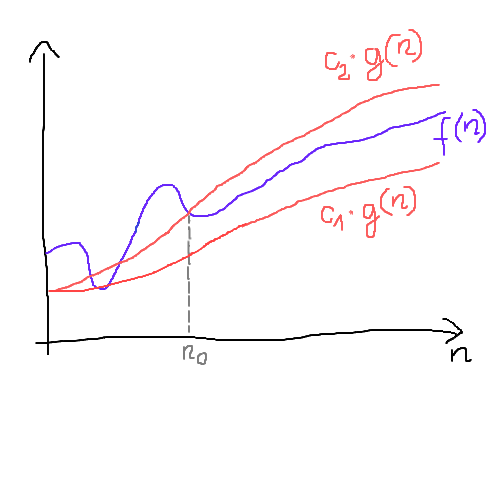## Bound Is Just a Bound

What’s the runtime complexity expressed in Big-O notation of this simple snippet below?

``````int foo(int[] numbers) {
int result = 0;
for (int i = 0; i < numbers.length; ++i) {
for (int j = 0; j < numbers.length; ++j) {
result +=  numbers[j];
}
}
return result;
}
``````

It is obviously O(n2). We have two nested loops and both of them iterate over the input array. What’s less obvious is that answers such as O(n3), O(2n) and even O(n!) are also correct. Take a look back at the definition of Big-O. These are all upper bounds of function n2. There is nothing wrong with saying that we are not more than 33 years old, when we are actually 25. It’s a true statement, however not very informative. We prefer having tight bounds rather than loose bounds. That’s why people are used to using as tight bounds as possible in Big-O notation. Nevertheless, if somebody asks you during an interview, what the time complexity of the algorithm, expressed in Big-O notation is, saying that it’s something very high such as O(n!) gives you quite good chance of being correct. However, I don’t really recommend it ;)

If Big-O notation can be so ambiguous, why do we use it? Why don’t we use Big-Θ instead of Big-O notation for all the algorithms we analyze? Wouldn’t it give us way more precise information? It seems that there are a couple of reasons for that. First of all, it’s very hard or even impossible to find a function which grows at exactly the same rate as the function we want to analyze. Have a look at this figure below (the function is defined for the integers n ≥ 1) and try to come up with an idea of what Big-Θ would it be?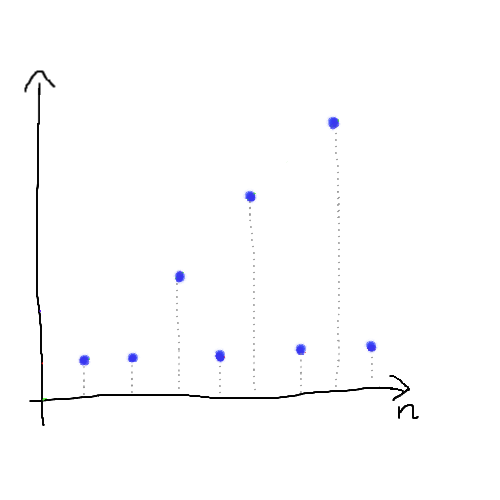It is not an easy task, is it? But let’s try to bound it from both sides instead.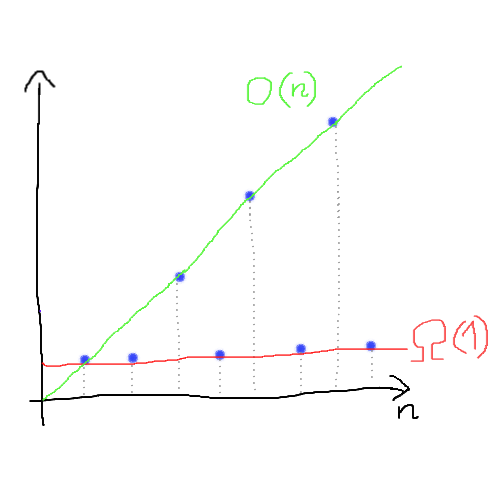We can easily find two simple linear and constant functions which bound our function f(n). Additionally, those are very tight bounds. Interested in what kind of algorithm would have this complexity? It can be actually something as simple as that:

``````foo(array of size n) {
if n is odd:
print("it's odd")
else:
array.forEach(print)
}``````

Another reason why Big-O notation is used more often is that people usually worry about what's the worst that can happen. In such cases, Big-O is sufficient, because it guarantees that it can't get much worse than what we estimated (it can still get a bit worse because we drop some less dominant factors).

But the first example with two nested loops is trivial — we can certainly say that it’s Θ(n2). It grows exactly at the same rate as the quadratic function. Similarly, the upper bound of the basic implementation of bubble sort is O(n2). In the worst case scenario, you can’t be better than that which means that it’s also Ω(n2). If we can find upper and lower bounds which are exactly the same, then we have our Big-Θ. So the runtime complexity of bubble sort is Ω(n2), however, in most sources, it is expressed as O(n2). Why is that? It seems that most people are more familiar with Big-O notation rather than Big-Θ. That’s why Big-O is a little bit abused in computer science.

## Difference Between Bound and Case

In the previous paragraph I mentioned that in the worst case scenario, bubble sort is Ω(n2). Why worst case scenario? Wouldn’t it grow slower if we consider the best case scenario? Yes, it would. But when we use asymptotic notation, unless stated otherwise, we are talking about worst-case running time.

I’ve noticed that a lot of people confuse lower (Big-Ω) bound with the best case scenario, upper bound (Big-O) with the worst case and Big-Θ with an average case. But these terms are not the same. If they were, then we wouldn't have the best-case performance for quicksort expressed as O(n log n), but Ω(n log n). It's important to remember that we can calculate any of Big-Ω, Big-O, or Big-Θ for best, average and the worst-case scenarios separately.

## Summary

It seems somehow obvious that algorithm which has asymptotically constant time is better than a linear or polynomial algorithm. But is it always like that? Just because an algorithm is Θ(1) doesn't mean it’s necessarily going to run faster than Θ(n2) algorithm for all sizes of n. Imagine Θ(1) algorithm with an enormous constant number of long-running operations like 1 billion. Then n parameter of Θ(n2) algorithm would have to grow extremely large before it’s slower than our Θ(1) algorithm. It’s important to keep in mind that asymptotic notation has its limitation and dropping some factors can potentially have a tremendous impact on algorithm performance in practice.

Topics:
big o ,complexity ,performance ,algorithm ,algorithmic

Comment (0)

Save
{{ articles.views | formatCount}} Views

Published at DZone with permission of

Opinions expressed by DZone contributors are their own.